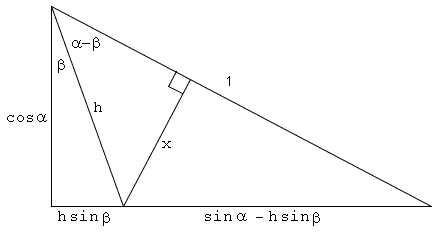# Addition and Subtraction Formulas for Sine and Cosine III

The proofs below of the addition and subtraction formulas for sine and cosine use the least amount of drawing attributes (5 lines each) and combine only three triangles all in an essential way. The proofs are by Leonard M. Smiley, University of Alaska Anchorage. A probably more professional copies of the proofs are available at the MAA site. They have also appeared in [R. Nelsen, pp. 42-43]. Naturally, the proofs are only valid for small angles, the constraints being clear from the diagrams. In fact, all four proofs use exactly same geometric configuration.

### Formulas for sin(α - β)h = cosα / cosβ. x = h sin(α - β). x = (sinα - h sinβ) cosα. sin(α - β) = sinα cos β - cos α sin β.

### Formulas for cos(α - β)h = cosα / sinβ. x = h cos(α - β). x = (sinα + h cosβ) cosα. cos(α - β) = cosα cos β + sin α sin β.

### Formulas for cos(α + β)sinαcosα
=
 cosβsinβ + cos(α+β)/sinα
cos(α + β) = cosα cos β - sin α sin β.

### Formulas for sin(α + β)sinα
=
 sin(α+β)cosβ + sinβ cosα / sinα
sin(α + β) = sinα cos β + cos α sin β.

### References

1. R. B. Nelsen, Proofs Without Words II, MAA, 2000### Trigonometry